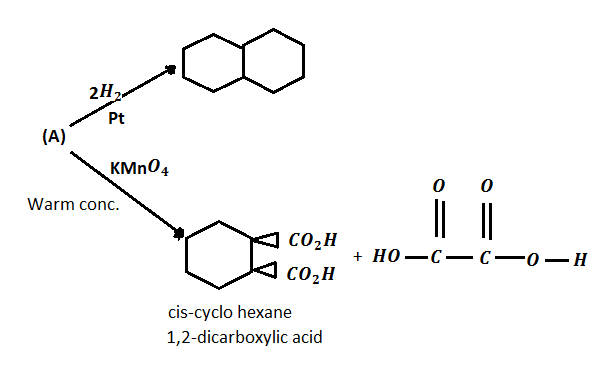Identify (A).

(a)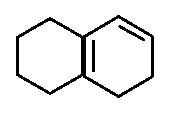(b)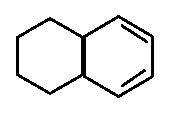(c)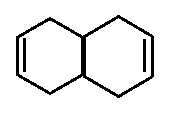(d)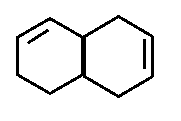High Yielding Test Series + Question Bank - NEET 2020

Difficulty Level: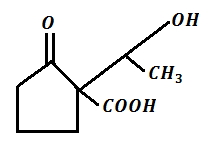Hence the product (Y) in the above sequence of reactions, is :

(a)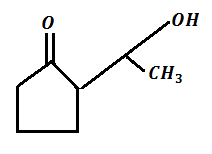(b)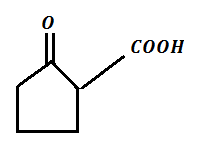(c)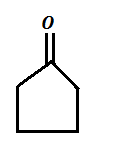(d)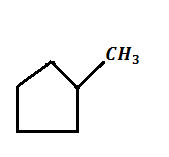Concept Questions :-

Preparation & properties of Aldehydes and Ketones
High Yielding Test Series + Question Bank - NEET 2020

Difficulty Level:

The action of bromine water (excess) on salicylic acid results in the formation of:-

(a)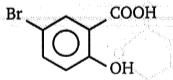(b)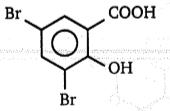(c)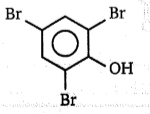(d)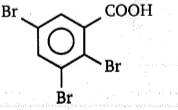Concept Questions :-

Preparation & properties of Aldehydes and Ketones
High Yielding Test Series + Question Bank - NEET 2020

Difficulty Level:

An organic compound having the molecular formula C8H10O on being heated with I2 and dilute NaOH gives a yellow precipitate. The expected compound is:

(a) C6H5CH2CH2OH

(b)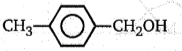(c)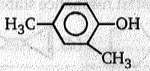(d)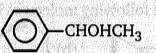Concept Questions :-

Preparation & properties of Aldehydes and Ketones
High Yielding Test Series + Question Bank - NEET 2020

Difficulty Level:

The Birch reduction of benzoic acid gives:-
(a)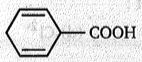(b)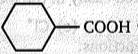(c)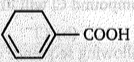(d)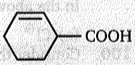Concept Questions :-

Preparation & properties of Aldehydes and Ketones
High Yielding Test Series + Question Bank - NEET 2020

Difficulty Level:

ph-CHO  +   2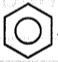$\stackrel{{\mathrm{H}}_{2}{\mathrm{SO}}_{4}}{\to }$major product of this reaction is:

(a) ph3CH

(b)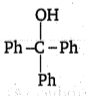(c)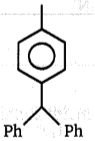(d) ph2CH2

Concept Questions :-

Preparation & properties of Aldehydes and Ketones
High Yielding Test Series + Question Bank - NEET 2020

Difficulty Level:

Which of the following acid gives positive Tollen's reagent test.

(a) $\left({\mathrm{CH}}_{2}{\right)}_{2}{<}_{{\mathrm{CO}}_{2}\mathrm{H}}^{{\mathrm{CO}}_{2}\mathrm{H}}$           (b)  ${\mathrm{CH}}_{2}{<}_{{\mathrm{CO}}_{2}\mathrm{H}}^{{\mathrm{CO}}_{2}\mathrm{H}}$            (c) $\underset{|}{\mathrm{C}}{\mathrm{O}}_{2}\mathrm{H}\phantom{\rule{0ex}{0ex}}{\mathrm{CO}}_{2}\mathrm{H}$              (d) HCO2H

Concept Questions :-

Preparation & properties of Aldehydes and Ketones
High Yielding Test Series + Question Bank - NEET 2020

Difficulty Level:

Acetaldehyde and benzaldehyde can be differentiated by:
(a) Fehling test
(b) Iodoform test
(c) Tollen's reagent
(d) both (a) and (b)

Concept Questions :-

Preparation & properties of Aldehydes and Ketones
High Yielding Test Series + Question Bank - NEET 2020

Difficulty Level:

When vapours of acetic acid are passed over.....at $300°\mathrm{C}$ we get acetone.

(a) ${\mathrm{Al}}_{2}{\mathrm{O}}_{3}$                        (b) $\mathrm{CuO}$

(c) $\mathrm{MoO}$                         (d) $\mathrm{Cu}$

Concept Questions :-

Preparation & properties of Aldehydes and Ketones
High Yielding Test Series + Question Bank - NEET 2020

Difficulty Level:

Formaldehyde is not used in: